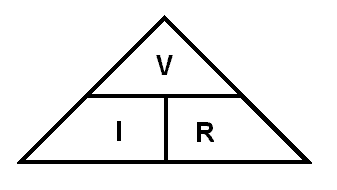RANDOM PAGE

SITE SEARCH

LOG
IN

HELP

# Ohm's Law

This is the AQA version closing after June 2019. Visit the the version for Eduqas instead.

## Definition of Resistance

Resistance ...

• opposes the free flow of current.
• is like a thin water pipe or a narrow road or a narrow doorway opposing the free flow of people.
• is measured in Ohms.
• is related to the current and voltage by Ohm's law.
• if a wire is replaced with a resistor, the current drops.

## Definition of the Ohm

• If the resistance is one Ohm, a potential difference of one Volt will cause a current of one Amp to flow.
• The Ohms tell you how many volts are needed to push one amp through a resistor. For example ...
• 10 Volts are needed to push one amp through a a 10 Ohm resistor.
• 2 Volts are needed to push one amp through a a 2 Ohm resistor.
• 1000000 Volts are needed to push one amp through a one megohm resistor. It would be interesting to watch the sparks fly if you could achieve this in real life!

## Ohm's Law

At a given temperature, the potential difference or Voltage drop (V) across a resistor (R) is proportional to the current (I) flowing through it.

## Calculations

R = V / I

• R = resistance in Ohms
• V = Voltage (potential difference) in Volts.
• I = current in Amps.

## Ohm's Law Triangle

This triangle helps some people to remember that

• R = V / I
• V = I R
• I = V / RTo remember this triangle, try this: The Vase on the table Is Red

The ammeter measures the current flowing through the resistor.

The voltmeter measures the voltage across the resistor.

Assume that the meters are ideal and they have no effect on the circuit ...

• No current flows through an ideal voltmeter.
• No voltage is lost across an ideal ammeter.

## Flash Practice

Try a few Ohm's Law calculations ...

reviseOmatic V3     Contacts, ©, Cookies, Data Protection and Disclaimers Hosted at linode.com, London